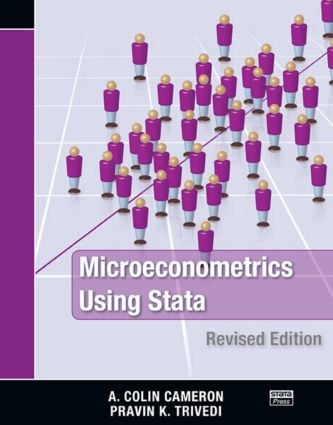Microeconometrics Using Stata

Revised Edition, 2nd Edition

Stata Press

706 pages

Purchasing Options:\$ = USD
Paperback: 9781597180733
pub: 2010-03-09
SAVE ~\$19.99
\$99.95
\$79.96
x

FREE Standard Shipping!

Description

A complete and up-to-date survey of microeconometric methods available in Stata, Microeconometrics Using Stata, Revised Edition is an outstanding introduction to microeconometrics and how to execute microeconometric research using Stata. It covers topics left out of most microeconometrics textbooks and omitted from basic introductions to Stata.

This revised edition has been updated to reflect the new features available in Stata 11 that are useful to microeconomists. Instead of using mfx and the user-written margeff commands, the authors employ the new margins command, emphasizing both marginal effects at the means and average marginal effects. They also replace the xi command with factor variables, which allow you to specify indicator variables and interaction effects. Along with several new examples, this edition presents the new gmm command for generalized method of moments and nonlinear instrumental-variables estimation. In addition, the chapter on maximum likelihood estimation incorporates enhancements made to ml in Stata 11.

Throughout the book, the authors use simulation methods to illustrate features of the estimators and tests described and provide an in-depth Stata example for each topic discussed. They also show how to use Stata’s programming features to implement methods for which Stata does not have a specific command. The unique combination of topics, intuitive introductions to methods, and detailed illustrations of Stata examples make this book an invaluable, hands-on addition to the library of anyone who uses microeconometric methods.

Stata Basics

Interactive use

Documentation

Command syntax and operators

Do-files and log files

Scalars and matrices

Using results from Stata commands

Global and local macros

Looping commands

Some useful commands

Template do-file

User-written commands

Data Management and Graphics

Introduction

Types of data

Inputting data

Data management

Manipulating datasets

Graphical display of data

Linear Regression Basics

Introduction

Data and data summary

Regression in levels and logs

Basic regression analysis

Specification analysis

Prediction

Sampling weights

OLS using Mata

Simulation

Introduction

Pseudorandom-number generators: Introduction

Distribution of the sample mean

Pseudorandom-number generators: Further details

Computing integrals

Simulation for regression: Introduction

GLS Regression

Introduction

GLS and FGLS regression

Modeling heteroskedastic data

System of linear regressions

Survey data: Weighting, clustering, and stratification

Linear Instrumental-Variables Regression

Introduction

IV estimation

IV example

Weak instruments

Better inference with weak instruments

3SLS systems estimation

Quantile Regression

Introduction

QR

QR for medical expenditures data

QR for generated heteroskedastic data

QR for count data

Linear Panel-Data Models: Basics

Introduction

Panel-data methods overview

Panel-data summary

Pooled or population-averaged estimators

Within estimator

Between estimator

RE estimator

Comparison of estimators

First-difference estimator

Long panels

Panel-data management

Linear Panel-Data Models: Extensions

Introduction

Panel IV estimation

Hausman–Taylor estimator

Arellano–Bond estimator

Mixed linear models

Clustered data

Nonlinear Regression Methods

Introduction

Nonlinear example: Doctor visits

Nonlinear regression methods

Different estimates of the VCE

Prediction

Marginal effects

Model diagnostics

Nonlinear Optimization Methods

Introduction

Newton–Raphson method

The ml command: lf method

Checking the program

The ml command: d0, d1, d2, lf0, lf1, and lf2 methods

The Mata optimize() function

Generalized method of moments

Testing Methods

Introduction

Critical values and p-values

Wald tests and confidence intervals

Likelihood-ratio tests

Lagrange multiplier test (or score test)

Test size and power

Specification tests

Bootstrap Methods

Introduction

Bootstrap methods

Bootstrap pairs using the vce(bootstrap) option

Bootstrap pairs using the bootstrap command

Bootstraps with asymptotic refinement

Bootstrap pairs using bsample and simulate

Alternative resampling schemes

The jackknife

Binary Outcome Models

Introduction

Some parametric models

Estimation

Example

Hypothesis and specification tests

Goodness of fit and prediction

Marginal effects

Endogenous regressors

Grouped data

Multinomial Models

Introduction

Multinomial models overview

Multinomial example: Choice of fishing mode

Multinomial logit model

Conditional logit model

Nested logit model

Multinomial probit model

Random-parameters logit

Ordered outcome models

Multivariate outcomes

Tobit and Selection Models

Introduction

Tobit model

Tobit model example

Tobit for lognormal data

Two-part model in logs

Selection model

Prediction from models with outcome in logs

Count-Data Models

Introduction

Features of count data

Empirical example 1

Empirical example 2

Models with endogenous regressors

Nonlinear Panel Models

Introduction

Nonlinear panel-data overview

Nonlinear panel-data example

Binary outcome models

Tobit model

Count-data models

Appendix A: Programming in Stata

Appendix B: Mata

Glossary

References

Author Index

Subject Index

Stata resources and Exercises appear at the end of each chapter.